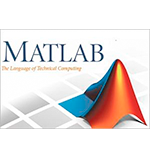# MATLAB介绍

MATLAB（矩阵实验室）是第四代高层次的编程语言和交互式环境数值计算，可视化和编程。

MATLAB是由MathWorks公司开发的。

• 矩阵和阵列处理

• 2-D和3-D绘图和图形

• 线性代数

• 代数方程组

• 非线性函数

• 统计

• 数据分析

• 微积分和微分方程

• 数值计算

• 积分

• 变换

• 曲线拟合

• 各种其它的特殊功能

## MATLAB的特点

• 数值计算，可视化和应用程序的开发，这是一个高层次的语言。

• 它还提供了一个互动的环境，探索，设计和解决问题的迭代。

• 它提供了广阔的线性代数，统计，傅立叶分析，筛选，优化，数值积分，解常微分方程的数学函数库。

• 它提供了内置的数据可视化工具，用于创建自定义绘制的图形。

• MATLAB的编程接口给开发工具，提高代码质量和可维护性和性能的最大化。

• 它提供了自定义的图形界面构建应用程序的工具。

• 它提供了基于MATLAB算法集成了C，Java，NET和Microsoft Excel等与外部应用程序和语言功能。

## MATLAB的用途

MATLAB被广泛用来作为计算工具在科学和工程涵盖领域物理，化学，数学和工程流。它在一定范围内的应用，包括：

• 信号处理和通信

• 图像和视频处理

• 控制系统

• 测试和测量

• 计算金融

• 计算生物学